# Test Prep Plan - Take a practice test

Take this practice test to check your existing knowledge of the course material. We'll review your answers and create a Test Prep Plan for you based on your results.
How Test Prep Plans work
1
2Based on your results, we'll create a customized Test Prep Plan just for you!
3Study smarter
Study more effectively: skip concepts you already know and focus on what you still need to learn.

# ASVAB Math: Working with Quadratic Equations Chapter Exam

Exam Instructions:

Choose your answers to the questions and click 'Next' to see the next set of questions. You can skip questions if you would like and come back to them later with the yellow "Go To First Skipped Question" button. When you have completed the practice exam, a green submit button will appear. Click it to see your results. Good luck!

### Page 1

#### Question 1 1. Find the solution to the equation below.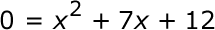### Page 2

#### Question 6 6. Expressing the equation below in the vertex form will give a value of 'h' = _____.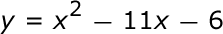### Page 3

#### Question 12 12. When completing the square for the quadratic equation shown below, how is c calculated?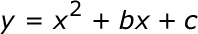#### Question 14 14. Find the value for c that must be added to both sides, and rewrite the equation in the vertex form.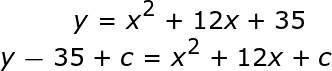#### Question 15 15. Factor and solve.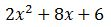### Page 4

#### Question 16 16. Factor.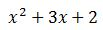#### Question 19 19. In order to complete the square on the following equation, what value would you add to both sides?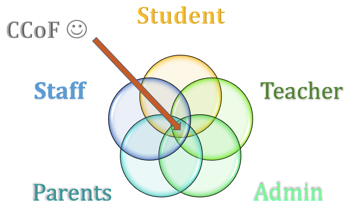#### Question 20 20. Factor and solve.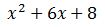### Page 5

#### Question 23 23. Factor.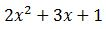#### Question 24 24. Factor and solve.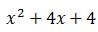#### Question 25 25. If the equation below told you a ball was 'h' feet in the air after 't' seconds, what would be the first step to finding out when it hit the ground?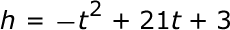### Page 6

#### Question 26 26. Rewrite the following equation in vertex form.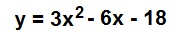#### Question 28 28. Find the solution to the equation below.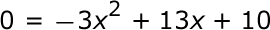#### ASVAB Math: Working with Quadratic Equations Chapter Exam Instructions

Choose your answers to the questions and click 'Next' to see the next set of questions. You can skip questions if you would like and come back to them later with the yellow "Go To First Skipped Question" button. When you have completed the practice exam, a green submit button will appear. Click it to see your results. Good luck!

Support